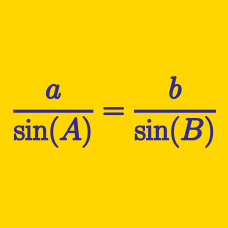Geometry

# Bearing Problems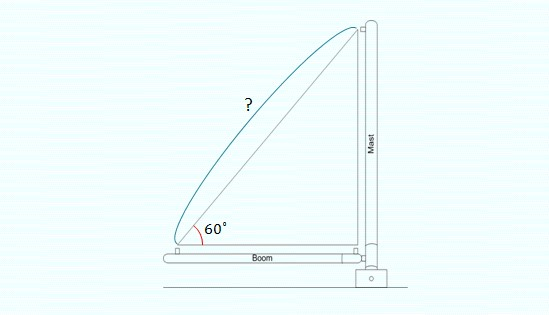The mast in the above diagram is $$9$$ meters high and a wire is stretched tight to form a straight line to the top of the mast at an angle of $$60^\circ.$$ How long is the wire in meters?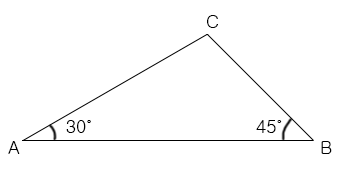The length of $$\overline{AC}$$ is 4 and the length of $$\overline{BC}$$ is $$2\sqrt{2}$$. What is the length of $$\overline{AB}$$?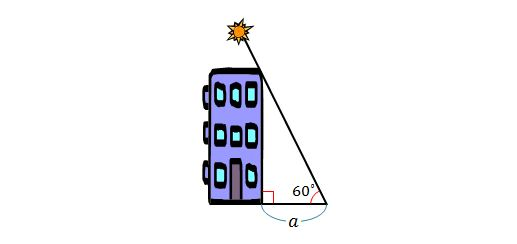In the above diagram, the length of the shadow of the building is $$a=32$$ meters and the end of the shadow forms an angle of $$60^\circ$$ with the sun. What is the height of the building?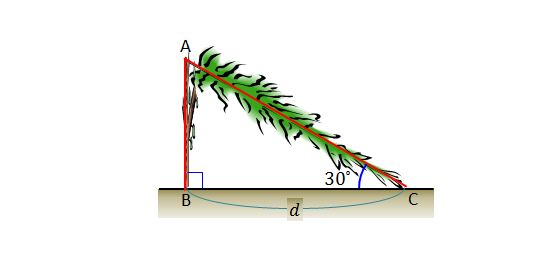A perfectly straight tree is struck by lightning and breaks at point $$A$$. After falling, the tree forms a triangle with the ground at angle $$\angle ACB =30^\circ$$ and the distance between the top and bottom of the tree is $$d =27\text{ m},$$ as illustrated in the above diagram. What is the height of the tree before being struck by lightning?The mast in the above diagram is $$21$$ meters high and a wire is stretched tight to form a straight line to the top of the mast at an angle of $$60^\circ.$$ How long is the wire in meters?

×

Problem Loading...

Note Loading...

Set Loading...